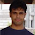### Young Alan Turing and the Arctangent

With the release of the new film "The Imitation Game", I decided to read the biography this excellent film was based on - Alan Turing: The Enigma. In it, the author Andrew Hodges relates the story that the 15-year-old Alan Turing derived the Maclaurin series for the $$\arctan$$ function, i.e. $\arctan(x) = x - \frac{x^3}{3} + \frac{x^5}{5} - \frac{x^7}{7} + \ldots$ This is trivial using calculus, but it's explicitly stated that young Alan Turing neither knew nor used calculus. How would you derived such a series without calculus?

This is a tricky problem, and I'd suggest first tackling the much easier problem of deriving the Maclaurin series for $$\exp(x)$$ from the relation $$\exp(2x) = \exp(x)\cdot \exp(x)$$. This is an underconstrained relation, so you'll need to assume $$c_0 = 1, c_1 = 1$$.

Getting back to $$\arctan$$, you could start with the half-angle formula for the tangent: $\tan(2x) = \frac{2\tan(x)}{1-{\tan}^2(x)}.$ Now use the Weierstrass-like substitution $$x = \arctan(t)$$ to get $\tan(2\arctan(t)) = \frac{2t}{1-t^2}.$ The right-hand side can be expanded in the usual geometric series fashion to get $\tan(2\arctan(t)) = 2t\cdot (1+t^2+t^4+\ldots).$
Finally, take the $$\arctan$$ of both sides and assume we have the series expansion $$\arctan(x) = c_1 x + c_3 x^3 + c_5 x^5 + \ldots$$. Note that we may ignore the terms with even powers of $$x$$ as $$\arctan(x)$$ is an odd function.

This gives us the setting $2\arctan(t) = \arctan(2t\cdot (1+t^2+t^4+\ldots))$ and expanding as a power series $2(c_1 t + c_3 t^3 + \ldots) = c_1 (2t\cdot (1+t^2+\ldots) + c_3 (2t\cdot (1+t^2+\ldots))^3 + \ldots$
The next step is to line up powers of $$t$$ on both sides and set up a system of simultaneous equations. There's some algebra and combinatorics involved, but we end up with the system of equations $c_{2i+1} = \sum_{j=0}^{i} c_{2j+1}\cdot 2^{2j} \cdot {{i+j} \choose {2j}}.$ Note that this system is underconstrained due to our functional relationship being satisfied by any multiple of the $$\arctan$$ function. We'll assume that $$c_1 = 1$$, but note that this follows from the classical (non-calculus) limit $$\lim_{x\to 0} \frac{\sin(x)}{x} = 1$$.

The first few relations are \begin{align*}
c_1 &= c_1 \\
c_3 &= c_1 + 4\cdot c_3 \\
c_5 &= c_1 + 12\cdot c_3 + 16\cdot c_5 \\
c_7 &= c_1 + 24\cdot c_3 + 80\cdot c_5 + 64\cdot c_7
\end{align*}
Assuming $$c_1 = 1$$ as above we quickly calculate $$c_3 = -\frac{1}{3}, c_5 = \frac{1}{5}, c_7 = -\frac{1}{7}$$, with the pattern being obvious.

That $$c_{2i+1} = \frac{(-1)^i}{2i+1}$$ can be verified by Wolfram Alpha:

An obvious question is whether or not there's a simple demonstration of this; in particular, one that a young Alan Turing may have found. This I don't know (yet).

1.Did you mean to link to the book 'Alan Turing: The Enigma' by Andrew Hodges?

http://www.amazon.com/Alan-Turing-Enigma-Inspired-Imitation-ebook/dp/B00M032W92/

1.Yes, thanks! Corrected.

### A Bayes' Solution to Monty Hall

For any problem involving conditional probabilities one of your greatest allies is Bayes' Theorem. Bayes' Theorem says that for two events A and B, the probability of A given B is related to the probability of B given A in a specific way.

Standard notation:

probability of A given B is written $$\Pr(A \mid B)$$
probability of B is written $$\Pr(B)$$

Bayes' Theorem:

Using the notation above, Bayes' Theorem can be written: $\Pr(A \mid B) = \frac{\Pr(B \mid A)\times \Pr(A)}{\Pr(B)}$Let's apply Bayes' Theorem to the Monty Hall problem. If you recall, we're told that behind three doors there are two goats and one car, all randomly placed. We initially choose a door, and then Monty, who knows what's behind the doors, always shows us a goat behind one of the remaining doors. He can always do this as there are two goats; if we chose the car initially, Monty picks one of the two doors with a goat behind it at random.

Assume we pick Door 1 and then Monty sho…

### Mixed Models in R - Bigger, Faster, Stronger

When you start doing more advanced sports analytics you'll eventually starting working with what are known as hierarchical, nested or mixed effects models. These are models that contain both fixed and random effects. There are multiple ways of defining fixed vs random random effects, but one way I find particularly useful is that random effects are being "predicted" rather than "estimated", and this in turn involves some "shrinkage" towards the mean.

Here's some R code for NCAA ice hockey power rankings using a nested Poisson model (which can be found in my hockey GitHub repository):
model <- gs ~ year+field+d_div+o_div+game_length+(1|offense)+(1|defense)+(1|game_id) fit <- glmer(model, data=g, verbose=TRUE, family=poisson(link=log) ) The fixed effects are year, field (home/away/neutral), d_div (NCAA division of the defense), o_div (NCAA division of the offense) and game_length (number of overtime periods); off…

### Gambling to Optimize Expected Median Bankroll

Gambling to optimize your expected bankroll mean is extremely risky, as you wager your entire bankroll for any favorable gamble, making ruin almost inevitable. But what if, instead, we gambled not to maximize the expected bankroll mean, but the expected bankroll median?

Let the probability of winning a favorable bet be $$p$$, and the net odds be $$b$$. That is, if we wager $$1$$ unit and win, we get back $$b$$ units (in addition to our wager). Assume our betting strategy is to wager some fraction $$f$$ of our bankroll, hence $$0 \leq f \leq 1$$. By our assumption, our betting strategy is invariant with respect to the actual size of our bankroll, and so if we were to repeat this gamble $$n$$ times with the same $$p$$ and $$b$$, the strategy wouldn't change. It follows we may assume an initial bankroll of size $$1$$.

Let $$q = 1-p$$. Now, after $$n$$  such gambles our bankroll would have a binomial distribution with probability mass function \[ \Pr(k,n,p) = \binom{n}{k} p^k q^{n-k…